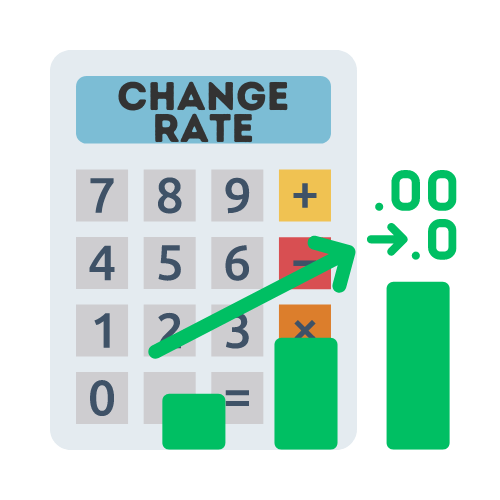# Rate of Change Calculator

## Calculate the average rate of change with this online calculator

Average Rate of Change:

View all Math Calculators

## What is the average rate of change?

The average rate of change is a number that quantifies how one value changes in relation to another. It's similar to slope, but it can be used for any function, not just linear ones. To calculate it, you take two points on the graph of the function and divide the change in y-value by the change in x-value.

## What is the Rate of Change?

Change is inevitable, and it is happening around us at all times. From the acceleration of your bike or car, to population growth, change is constant. And while some changes can be predicted, others can take us by surprise. Change can be difficult to adapt to, but it is also what keeps life interesting.

The rate of change allows us to measure the rate at which something is changing. This can be useful in a variety of situations. It can be used to:

• measure the rate of change in economic growth
• measure the rate of change in a population
• measure the rate of change in diseases
• measure the rate of change in the cost of living

## Why is the Rate of Change Important?

The rate of change is important in different fields, because it is a measure of how fast something is changing. This information can be used to make predictions about the future.

For example, if the rate of change in the stock market is increasing, we can predict that the stock prices will continue to rise. If the rate of change in the temperature is increasing, we can predict that the weather will continue to get warmer.

### The Rate of Change in Business

In the business world, the rate of change can be a critical indicator of a company's health and future prospects. A company that is growing quickly may be able to take advantage of opportunities and expand its market share, while a company that is growing slowly may be at risk of losing market share to its competitors.

### The Rate of Change in Investments

In the world of investing, the rate of change is also important. An investor looking at a company's stock price may want to know how the stock has performed over time, and the rate of change is one way to measure this. An investor looking at a company's financial statements may want to know how the company's revenue and expenses have changed over time, and the rate of change is again one way to measure this.

### The Rate of Change in Physics

In the world of physics, the rate of change is important in many calculations. For example, the rate of change of velocity is used to calculate acceleration. The rate of change of position is used to calculate velocity. And the rate of change of a function is used to calculate its derivative.

## How to Calculate the Average Rate of Change

If you want to know how to measure rate of change manually, just follow these 3 easy steps:

1. Measure the coordinate points of point 1 (example: 1,2)
2. Measure the coordinate points of point 2 (example: 3,6)
3. Use rate of change formula to calculate: `(6-3/2-1) = 3/1 = 3`

You can also calculate rate of change by using our rate of change calculator (above).

## What is the Rate of Change Formula?

The average rate of change formula can be written as:
`Rate of Change = (y₂ - y₁) / (x₂ - x₁)`

Sometimes you may hear rate of change of a line being referred to as the slope, or rise over run.

You can find the rate of change of a line by using a similar formula and substituting x and y. This will give you the rate of change of x with respect to y, or run over rise.

There are also similar alternatives to using this calculator. For example, the percentage change calculator is useful in measuring the change in two values.

## Rate of Change Example

For this example, we will calculate the rate of change for height (inches) based on age (years), using the table below:

Solution:
We can calculate rate of change using the rate of change formula:

Rate of change = (change in column 1) / (change in column 2)

In this example we can summarize this as:
Rate of change = (change in inches) / (change in years)

Rate of change = (54-40) / (10-5)
Rate of change = 14 / 5
Rate of change = 2.8

Answer: The rate of change is 2.8 inches per year.